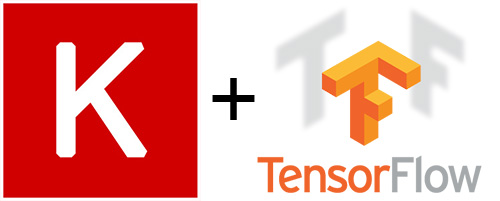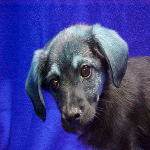# An Easy Guide to build new TensorFlow Datasets and Estimator with Keras ModelTensorFlow r1.4 was release not long ago late October.

If you haven't updated yet,

`pip3 install --upgrade tensorflow-gpu`

Some changes worth notice,

• Keras is now part of the core TensorFlow package
• Dataset API become part of the core package
• Some enhancements to the Estimator allow us to turn Keras model to TensorFlow estimator and leverage its Dataset API.

In this post, I will show you how to turn a Keras image classification model to TensorFlow estimator and train it using the Dataset API to create input pipelines.

If you haven't read TensorFlow team's Introduction to TensorFlow Datasets and Estimators post. Read it now to have an idea why we do what we do here.

See you just happen to be in a region where you do not have access to any Google's websites, which kindly sucks, so I summarized it here for you.

## Summarized Intro to TensorFlow Datasets API and Estimators

### Datasets API

You should use Dataset API to create input pipelines for TensorFlow models. It is the best practice way because:

• The Dataset API provides more functionality than the older APIs (`feed_dict` or the queue-based pipelines).
• It performs better.
• It is cleaner and easier to use.
• Its pipeline for an image model might aggregate data from files in a distributed file system, apply random perturbations to each image, and merge randomly selected images into a batch for training.
• I also have pipeline for text model

### Estimators

Estimators is a high-level API that reduces much of the boilerplate code you previously needed to write when training a TensorFlow model.

Two possible way to create Estimators: Pre-made Estimators to generate a specific type of model, and the other one is to create your own with its base class.

Keras integrates smoothly with other core TensorFlow functionality, including the Estimator API

All right, enough for the intros, let's get to the point to build our Keras Estimator.

## Get down to the code

For simplicity reason, let's build a classifier for the famous dog vs cat image classification.

The cats vs. dogs dataset was made available by Kaggle.com as part of a computer vision competition in late 2013. You can download the original dataset at https://www.kaggle.com/c/dogs-vs-cats/download/train.zip

We are only using a small portion of the training data.

After downloading and uncompressing it, we will create a new dataset containing three subsets: a training set with 1000 samples of each class, and a test set with 500 samples of each class. This part of the code is omitted here, check out my GitHub to grab it.

### Build Keras model

```from tensorflow.python.keras.applications.vgg16 import VGG16
from tensorflow.python.keras import models
from tensorflow.python.keras import layers

conv_base = VGG16(weights='imagenet',
include_top=False,
input_shape=(150, 150, 3))

model = models.Sequential()
conv_base.trainable = False
model.compile(loss='binary_crossentropy',
optimizer=tf.keras.optimizers.RMSprop(lr=2e-5),
metrics=['acc'])
```

We are leveraging the pre-trained VGG16 model's convolution layers. aka the "convolutional base" of the model. Then we add our own classifier fully connected layers to do binary classification(cat vs dog).

Note that since we don't want to touch the parameters pre-trained in the "convolutional base", so we set them as not trainable. Want to go deeper how this model works? Check out this great jupyter notebook by the creator of Keras.

### Keras model to TensorFlow Estimator

```model_dir = os.path.join(os.getcwd(), "models//catvsdog").replace("//", "\\")
os.makedirs(model_dir, exist_ok=True)
print("model_dir: ",model_dir)
est_catvsdog = tf.keras.estimator.model_to_estimator(keras_model=model,
model_dir=model_dir)
```

model_dir will be our location to store trained tensorflow models. Training progress can be viewed by TensorBoard.
I found that I have to specify the full path, otherwise, otherwise Tensorflow will complain about it later during training.

### Image Input function

When we train our model, we'll need a function that reads the input image files/labels and returns the image data and labels. Estimators require that you create a function of the following format:

```def input_fn():
...<code>...
return ({ 'input_1':[ImagesValues]},
[ImageTypeLogits])
```

The return value must be a two-element tuple organized as follows: :

- The first element must be a dictionary in which each input feature is a key. We have only one 'input_1' here which is the input layer name for the model that took processed image data as input for the training batch.
- The second element is a list of labels for the training batch.

So here is important code that makes the input function for our model.

```def imgs_input_fn(filenames, labels=None, perform_shuffle=False, repeat_count=1, batch_size=1):
def _parse_function(filename, label):
image = tf.image.decode_image(image_string, channels=3)
image.set_shape([None, None, None])
image = tf.image.resize_images(image, [150, 150])
image = tf.subtract(image, 116.779) # Zero-center by mean pixel
image.set_shape([150, 150, 3])
image = tf.reverse(image, axis=) # 'RGB'->'BGR'
d = dict(zip([input_name], [image])), label
return d
if labels is None:
labels = *len(filenames)
labels=np.array(labels)
# Expand the shape of "labels" if necessory
if len(labels.shape) == 1:
labels = np.expand_dims(labels, axis=1)
filenames = tf.constant(filenames)
labels = tf.constant(labels)
labels = tf.cast(labels, tf.float32)
dataset = tf.data.Dataset.from_tensor_slices((filenames, labels))
dataset = dataset.map(_parse_function)
if perform_shuffle:
# Randomizes input using a window of 256 elements (read into memory)
dataset = dataset.shuffle(buffer_size=256)
dataset = dataset.repeat(repeat_count)  # Repeats dataset this # times
dataset = dataset.batch(batch_size)  # Batch size to use
iterator = dataset.make_one_shot_iterator()
batch_features, batch_labels = iterator.get_next()
return batch_features, batch_labels
```

Arguments for this function

• filenames, an array of image file names
• labels=None, an array of the image labels for the model. Set to None for inference
• perform_shuffle=False, useful when training, read batch_size records, then shuffles (randomizes) their order.
• repeat_count=1, useful when training, repeat the input data several times for each epoch
• batch_size=1, reads batch_size records at a time

As a sanity check, let's dry run the imgs_input_fn() and look at its output.

```next_batch = imgs_input_fn(test_files, labels=test_labels, perform_shuffle=True, batch_size=20)
with tf.Session() as sess:
first_batch = sess.run(next_batch)
x_d = first_batch['input_1']

print(x_d.shape)
img = image.array_to_img(x_d)
img.show()
```

It output the shape of our image and image itself

`(20, 150, 150, 3)`Looks like color channels 'RGB' has changed to 'BGR' and shape resized to (150, 150) for our model. That is the correct input format the VGG16's "convolutional base" is expecting.

### Train and Evaluate

TensorFlow release 1.4 also introduces the utility function `tf.estimator.train_and_evaluate` which simplifies training, evaluation and exporting Estimator models.

This function enables distributed execution for training and evaluation, while still supporting local execution.

```train_spec = tf.estimator.TrainSpec(input_fn=lambda: imgs_input_fn(test_files,
labels=test_labels,
perform_shuffle=True,
repeat_count=5,
batch_size=20),
max_steps=500)
eval_spec = tf.estimator.EvalSpec(input_fn=lambda: imgs_input_fn(test_files,
labels=test_labels,
perform_shuffle=False,
batch_size=1))

tf.estimator.train_and_evaluate(est_catvsdog, train_spec, eval_spec)
```

The model training result will be saved to ./models/catvsdog directory. If you are interested, you can take a look at the summary in TensorBoard

`tensorboard --logdir=./models/catvsdog`

### Predict

Here we only predict the first 10 images in the test_files.

```predict_results = est_catvsdog.predict(
input_fn=lambda: imgs_input_fn(test_files[:10],
labels=None,
perform_shuffle=False,
batch_size=10))
predict_logits = []
for prediction in predict_results:
predict_logits.append(prediction['dense_2'])
```

To predict we can set the labels to None because that is what we will be predicting. 'dense_2' is our model's output layer name, `prediction['dense_2']` will be one single float number between 0~1 where 0 means a cat image and 1 is a dog image.

Check the prediction result

```predict_is_dog = [logit > 0.5 for logit in predict_logits]
actual_is_dog = [label > 0.5 for label in test_labels[:10]]
print("Predict dog:",predict_is_dog)
print("Actual dog :",actual_is_dog)
```

It outputs

```Predict dog: [False, False, True, False, True, True, False, False, False, False]
Actual dog : [False, False, True, False, True, True, False, False, False, False]```

The model correctly classified all 10 images.

## Summary

We build a Keras Image classifier, turn it into a TensorFlow Estimator, build the input function for the Datasets pipeline. Finally, train and estimate the model. Go ahead and check out the full source code in my GitHub repo for this post.

Introduction to TensorFlow Datasets and Estimators-Google developers blog

Announcing TensorFlow r1.4 -Google developers blog

TensorFlow r1.40 release note

Datasets API guide

Estimators API guide

Current rating: 2.7